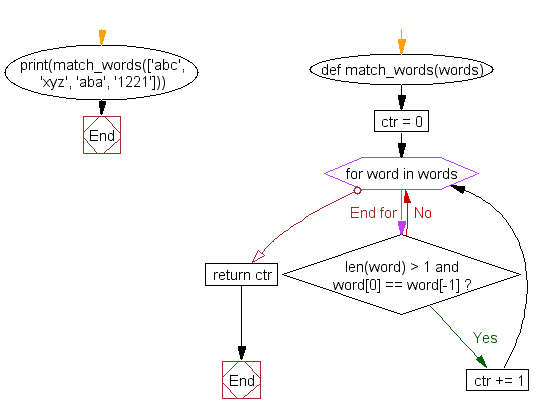﻿ Python: Count the number of strings where the string length is 2 or more and the first and last character are same from a given list of strings - w3resource# Python: Count the number of strings where the string length is 2 or more and the first and last character are same from a given list of strings

## Python List: Exercise-5 with Solution

Write a Python program to count the number of strings where the string length is 2 or more and the first and last character are same from a given list of strings.

Sample Solution:-

Python Code:

``````def match_words(words):
ctr = 0

for word in words:
if len(word) > 1 and word == word[-1]:
ctr += 1
return ctr

print(match_words(['abc', 'xyz', 'aba', '1221']))
```
```

Sample Output:

```2
```

Flowchart:## Visualize Python code execution:

The following tool visualize what the computer is doing step-by-step as it executes the said program:

Python Code Editor:

Have another way to solve this solution? Contribute your code (and comments) through Disqus.

What is the difficulty level of this exercise?

Test your Python skills with w3resource's quiz

﻿

## Python: Tips of the Day

Floor Division:

When we speak of division we normally mean (/) float division operator, this will give a precise result in float format with decimals.

For a rounded integer result there is (//) floor division operator in Python. Floor division will only give integer results that are round numbers.

```print(1000 // 300)
print(1000 / 300)```

Output:

```3
3.3333333333333335```